# The Augmented Pentagonal Prism

The augmented pentagonal prism (J52) is the 52nd Johnson solid. It has 11 vertices, 19 edges, and 10 polygons (4 triangles, 4 squares, and 2 pentagons).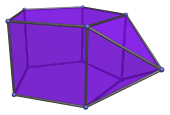It can be constructed augmenting a pentagonal prism with a square pyramid. A second square pyramid can be added in one of the non-adjacent positions of the prism to make a biaugmented pentagonal prism (J53).

## Projections

Here are some views of the augmented pentagonal prism from various angles:

Projection Description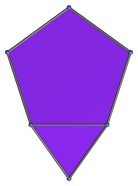Top view.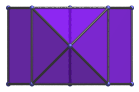Front view.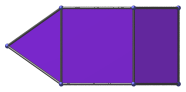Side view.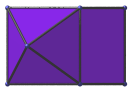72° rotated side view.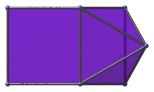144° rotated side view.

## Coordinates

The Cartesian coordinates of the augmented pentagonal prism, centered on the origin and with edge length 2, are:

• (0, √((10+2√5)/5), ±1)
• (±φ, √((5−√5)/10), ±1)
• (±1, −√((5+2√5)/5), ±1)
• (0, −(√2 + √((5+2√5)/5)), 0)

where φ=(1+√5)/2 is the Golden Ratio.

Last updated 19 Jun 2018.